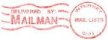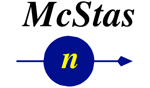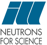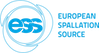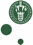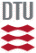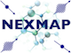McStas: Lens_simple Component

# The `Lens_simple` Component

Rectangular/circular slit with parabolic/spherical LENS.

## Identification

• Author: Henrich Frielinghaus
• Origin: FZ Juelich
• Date: June 2010
• Version:(Unknown)

## Description

```A simple rectangular or circular slit. You may either
specify the radius (circular shape), or the rectangular bounds.
No transmission around the slit is allowed.

At the slit position there is/are also a LENS or multiple LENSES present.
Spherical/parabolic abberation is taken into account in a simplified
manner. The focal point becomes a function of the distance ss from the
origin of the lens where the ray hits the lens (plane).
With this focal distance the refraction is calculated based on simple
geometrical optics (as tought already in school).

The approximation gets wrong when the distance ss is too big, and
total reflection becomes possible. Then the corrections of the focal
distance are strong (see foc*= ... lines. When this correction factor
is too close to zero, the corrections become highly wrong).

The advantage of this routine is the simplicity which makes the
simulation very fast - especially for multiple lenses. The argument
for this way of treating the lenses is that the collimation and
detector distance are large (say 20m) compared to the lens thickness
(say 2-5cm) and the lens array thickness (say max. 1m).

For lens arrays one still can simulate several of these simplified
lenses instead of an exact treatment (because 5cm<<1m). The author
believes that this medium detailed treatment meets usually the
desired accuracy.

Example: Lens_simple(rho=5.16e10,Rc=0.0254,Nl=7,xmin=-0.01,xmax=0.01,ymin=-0.01,ymax=0.01)
^^^^^^^^^^^ last example includes absorption of MgF2-lens.

```
WARNING: This is a contributed Component.

## Input parameters

Parameters in boldface are required; the others are optional.
Name Unit Description Default
rho m-2 Scattering length density 5.16e14
Rc m Radius (concave: Rc>0) of biconcave lens 0.02
Nl 1 Number of single lenses 7.0
parab not 0 -> parabolic, for 0 spherical [] Switch 1.1
xmin m Lower x bound 0
xmax m Upper x bound 0
ymin m Lower y bound 0
ymax m Upper y bound 0
radius m Radius of slit in the z=0 plane, centered at Origin 0
SigmaAL 1/(m*AA) Absorption cross section per lambda (wavelength) 0.141
d0 m Minimum thickness in the centre of the lens 0.002